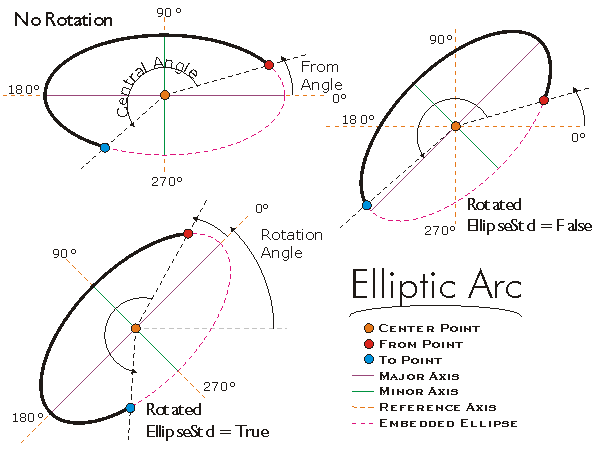This document is archived and information here might be outdated.  Recommended version.

IEllipticArc.ToAngle Property (ArcObjects .NET 10.7 SDK)
 ArcObjects Help for .NET developers > ArcObjects Help for .NET developers > ArcObjects namespaces > Geometry > ESRI.ArcGIS.Geometry > Interfaces > IE > IEllipticArc Interface > IEllipticArc.ToAngle Property
 ArcGIS Developer Help

# IEllipticArc.ToAngle Property

The end angle (measured from a horizontal line through the center point) defining where the arc ends.

```[Visual Basic .NET]
Public Function get_ToAngle ( _
ByVal ellipseStd As Boolean _
) As Double
```
```[Visual Basic .NET]
Public Sub set_ToAngle ( _
ByVal ellipseStd As Boolean, _
ByVal ToAngle As Double _
)
```
```[C#]
public double get_ToAngle (
bool ellipseStd
);
```
```[C#]
public void set_ToAngle (
bool ellipseStd,
double ToAngle
);
```
```[C++]
HRESULT get_ToAngle(
VARIANT_BOOL ellipseStd,
System.Double* ToAngle
);
```
```[C++]
HRESULT put_ToAngle(
VARIANT_BOOL ellipseStd,
double ToAngle
);
```
```[C++]
Parameters
ellipseStd
ellipseStd is a parameter of type bool
ToAngle [out, retval]
ToAngle is a parameter of type double*
ellipseStd
ellipseStd is a parameter of type bool
ToAngle
ToAngle is a parameter of type double
```

#### Product Availability

Available with ArcGIS Engine, ArcGIS Desktop, and ArcGIS Server.

#### Description

The To Angle is the angle of the Line defined by the Center Point and the To Point of the Elliptic Arc.  Setting the To Angle changes the To Point of the Elliptic Arc without altering the embedded geometry.  The To Angle is measured in radians (there are 2*pi radians in a full ellipse).  If EllipseStd = TRUE, the To Angle is relative to the rotated semi-Major Axis rather than the X-Axis.

#### Remarks#### See Also

IEllipticArc Interface